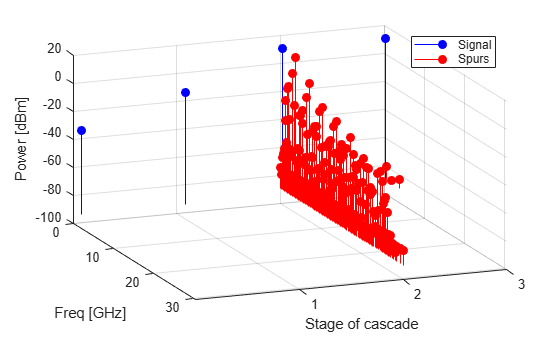Documentation

## Visualizing Mixer Spurs

This example shows how to create an `rfckt.mixer` object and plot the mixer spurs of that object.

Mixers are non-linear devices used in RF systems. They are typically used to convert signals from one frequency to another. In addition to the desired output frequency, mixers also produce intermodulation products (also called mixer spurs), which are unwanted side effects of their nonlinearity. The output of the mixer occurs at the frequencies:

`${F}_{out}\left(N,M\right)=|N{F}_{in}+M{F}_{LO}|$`

where:

• ${F}_{in}$ is the input frequency.

• ${F}_{LO}$ is the local oscillator (LO) frequency.

• $N$ is a nonnegative integer.

• $M$ is an integer.

Only one of these output frequencies is the desired tone. For example, in a downconversion mixer (i.e. ${F}_{in}={F}_{RF}$) with a low-side LO (i.e. ${F}_{RF}>{F}_{LO}$), the case $N=1$, $M=-1$ represents the desired output tone. That is:

`${F}_{out}\left(1,-1\right)={F}_{IF}=|N{F}_{in}+M{F}_{LO}|={F}_{RF}-{F}_{LO}$`

All other combinations of $N$ and $M$ represent the spurious intermodulation products.

Intermodulation tables (IMTs) are often used in system-level modeling of mixers. This example first examines the IMT of a mixer. Then the example reads an `.s2d` format file containing an IMT, and plots the output power at each output frequency, including the desired signal and the unwanted spurs. The example also creates a cascaded circuit which contains a mixer with IMT followed by a filter, whose purpose is to mitigate the spurs, and plots the output power before and after mitigation.

For more information on IMTs, see the OpenIF example Finding Free IF Bandwidths.

### Create a Mixer Object from a Data File

Create an `rfckt.mixer` object to represent the downconverting mixer that is specified in the file, `samplespur1.s2d`. The mixer is characterized by S-parameters, spot noise and IMT. These data are stored in the `NetworkData`, `NoiseData` and `MixerSpurData` properties of the `rfckt` object, respectively.

```Mixer = rfckt.mixer('FLO', 1.7e9); % Flo = 1.7GHz read(Mixer,'samplespur1.s2d'); disp(Mixer)```
``` rfckt.mixer with properties: MixerSpurData: [1x1 rfdata.mixerspur] MixerType: 'Downconverter' FLO: 1.7000e+09 FreqOffset: [] PhaseNoiseLevel: [] NoiseData: [1x1 rfdata.noise] NonlinearData: Inf IntpType: 'Linear' NetworkData: [1x1 rfdata.network] nPort: 2 AnalyzedResult: [1x1 rfdata.data] Name: 'Mixer' ```
`IMT = Mixer.MixerSpurData.data`
```IMT = 16×16 99 26 35 39 50 41 53 49 51 42 62 51 60 47 77 50 24 0 35 13 40 24 45 28 49 33 53 42 60 47 63 99 73 73 74 70 71 64 69 64 69 62 74 62 72 60 99 99 67 64 69 50 77 47 74 44 74 47 75 44 70 99 99 99 86 90 86 88 88 85 86 85 90 85 85 85 99 99 99 99 90 80 90 71 90 68 90 65 88 65 85 99 99 99 99 99 90 90 90 90 90 90 90 90 90 90 99 99 99 99 99 99 90 90 90 90 90 87 90 90 90 99 99 99 99 99 99 99 99 95 99 95 99 95 99 95 99 99 99 99 99 99 99 99 90 95 90 90 90 99 90 99 99 99 99 99 99 99 99 99 ⋮ ```

### Plot the Mixer Output Signal and Spurs

Use the `plot` method of the `rfckt` object to plot the power of the desired output signal and the spurs. The second input argument must be the string `'MIXERSPUR'`. The third input argument must be the index of the circuit for which to plot output power data. The `rfckt.mixer` object only contains one circuit (the mixer), so index 0 corresponds to the mixer input and index 1 corresponds to the mixer output.

```CktIndex = 1; % Plot the output only Pin = -10; % Input power is -10dBm Fin = 2.1e9; % Input frequency is 2.1GHz figure plot(Mixer,'MIXERSPUR',CktIndex,Pin,Fin);```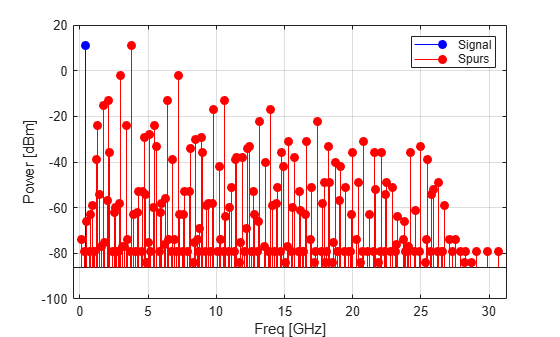### Use the Data Cursor

Run the cursor over the plot to get the frequency and power level of each signal and spur.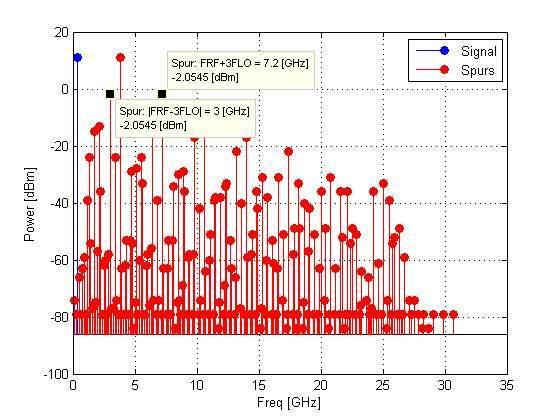Create an amplifier object for LNA, mixer, and LC Bandpass Tee objects. Then build the cascade shown in the following figure: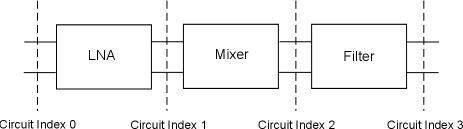```FirstCkt = rfckt.amplifier('NetworkData', ... rfdata.network('Type','S','Freq',2.1e9,'Data',[0,0;10,0]), ... 'NoiseData',0,'NonlinearData',Inf); % 20dB LNA SecondCkt = copy(Mixer); % Mixer with IMT table ThirdCkt = rfckt.lcbandpasstee('L',[97.21 3.66 97.21]*1.0e-9, ... 'C',[1.63 43.25 1.63]*1.0e-12); % LC Bandpass filter CascadedCkt = rfckt.cascade('Ckts',{FirstCkt,SecondCkt,ThirdCkt});```

### Plot the Output Signal and Spurs of the LC filter in a Cascade

Use the `plot` method of the `rfckt` object to plot the power of the desired output signal and the spurs. The third input argument is `3`, which directs the toolbox to plot the power at the output of the third component of the cascade (the LC filter).

```CktIndex = 3; % Plot the output signal and spurs of the LC filter, % which is the 3rd circuit in the cascade Pin = -30; % Input power is -30dBm Fin = 2.1e9; % Input frequency is 2.1GHz plot(CascadedCkt,'MIXERSPUR',CktIndex,Pin,Fin)```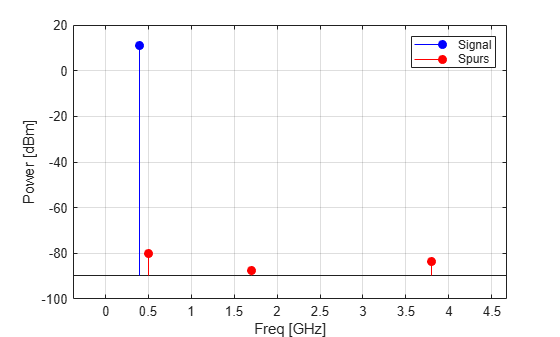### Plot the Cascade Signal and Spurs in 3D

Use the `plot` method of the `rfckt` object with a third input argument of `'all'` to plot the input power and the output power after each circuit component in the cascade. Circuit index 0 corresponds to the input of the cascade. Circuit index 1 corresponds to the output of the LNA. Circuit index 2 corresponds to the output of the mixer, which was shown in the previous plot. Circuit index 3 corresponds to the output of the LC Bandpass Tee filter.

```CktIndex = 'all'; % Plot the input signal, the output signal, and the % spurs of the three circuits in the cascade: FirstCkt, % SecondCkt and ThirdCkt Pin = -30; % Input power is -30dBm Fin = 2.1e9; % Input frequency is 2.1GHz plot(CascadedCkt,'MIXERSPUR',CktIndex,Pin,Fin) view([68.5 26])```